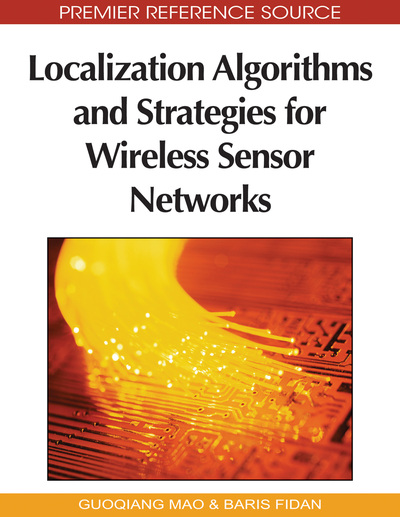# Machine Learning Based Localization

Duc A. Tran (University of Massachusetts, USA), XuanLong Nguyen (Duke University, USA) and Thinh Nguyen (Oregon State University, USA)
DOI: 10.4018/978-1-60566-396-8.ch012

## Abstract

A vast majority of localization techniques proposed for sensor networks are based on triangulation methods in Euclidean geometry. They utilize the geometrical properties of the sensor network to infer the sensor locations. A fundamentally different approach is presented in this chapter. This approach is based on machine learning, in which the authors work directly on the natural (non-Euclidean) coordinate systems provided by the sensor devices. The known locations of a few nodes in the network and the sensor readings can be exploited to construct signal-strength or hop-count based function spaces that are useful for learning unknown sensor locations, as well as other extrinsic quantities of interest. They discuss the applicability of two learning methods: the classification method and the regression method. They show that these methods are especially suitable for target tracking applications.
Chapter Preview
Top

## Introduction

A sensor node knows its location either via a built-in GPS-like device or a localization technique. A straightforward localization approach is to gather the information (e.g., connectivity, pair-wise distance measure) about the entire network into one place, where the collected information is processed centrally to estimate the nodes’ locations using mathematical algorithms such as Semidefinite Programming (Doherty et al. (2001)) and Multidimensional Scaling (Shang et al. (2003)).

Many techniques attempt localization in a distributed manner. The relaxation-based techniques (Savarese et al. (2001), Priyantha et al. (2003)) start with all the nodes in initially random positions and keep refining their positions using algorithms such as local neighborhood multilateration and convex optimization. The coordinate-system stitching techniques (Capkun et al. (2001), Meertens & Fitzpatrick (2004), Moore et al. (2004)) divide the network into overlapping regions, nodes in each region being positioned relatively to the region's local coordinate system (a centralized algorithm may be used here). The local coordinate systems are then merged, or “stitched”, together to form a global coordinate system. Localization accuracy can be improved by using a set of nodes with known locations, called the beacon nodes, and extrapolate unknown node locations from the beacon locations (Bulusu et al. (2002), Savvides et al. (2001), Savvides et al. (2002), Niculescu & Nath (2003a), Nagpal et al. (2003), He et al. (2003)).

Most current techniques assume that the distance between two neighbor nodes can be measured, typically via a ranging procedure. In this procedure, various information can be used to help estimate pair-wise distance, such as Received Signal Strength Indication (RSSI) (Whitehouse (2002)), Time Difference of Arrival (TDoA) (Priyantha (2005), Kwon et al. (2004)), or Angle of Arrival (AoA) (Priyantha et al. (2001), Niculescu & Nath (2003a)). Other range measurement methods can be found in (Priyantha (2001b), Savvides et al. (2001b), Priyantha (2005b), Lee & Scholtz (2002), Gezici et al. (2005)).

To avoid the cost of ranging, range-free techniques have been proposed (Bulusu et al. (2002), Meertens & Fitzpatrick (2004), He et al. (2003), Stoleru et al. (2005), Priyantha et al. (2005)). APIT (He et al. (2003)) assumes that a node can hear from a large number of beacons. Spotlight (Stoleru et al. (2005)) requires an aerial vehicle to generate light onto the sensor field. (Priyantha et al. (2005)) uses a mobile node to assist pair-wise distance measurements until a “global rigid” state can be reached where the sensor locations can be uniquely determined. DV-Hop (Niculescu & Nath (2003b)) and Diffusion (Bulusu et al. (2002), Meertens & Fitzpatrick (2004)) are localization techniques requiring neither ranging nor external assisting devices.

All the aforementioned techniques use Euclidean geometrical properties to infer the sensor nodes’ locations. Recently, a number of techniques that employ the concepts from machine learning have been proposed (Brunato & Battiti (2005), Nguyen et al. (2005), Pan et al. (2006), Tran & Nguyen (2006), Tran & Nguyen (2008), Tran & Nguyen (2008b)). The main insight of these methods is that the topology implicit in sets of sensor readings and locations can be exploited in the construction of possibly non-Euclidean function spaces that are useful for the estimation of unknown sensor locations, as well as other extrinsic quantities of interest. Specifically, one can assume a set of beacon nodes and use them as the training data for a learning procedure. The result of this procedure is a prediction model that will be used to localize the sensor nodes of previously unknown positions.

## Complete Chapter List

Search this Book:
Reset